This video was brought to you by GeneralPAC.com, making power systems Intuitive, Open and Free for Everyone, Everywhere. Consider subscribing and supporting through patreon.com/GeneralPAC. This is a mechanism for you to support us financially so we can continue making high quality power system video tutorials. Our corporate sponsor for this topic is AllumiaX.com from Seattle, Washington. Contact them for industrial and commercial power system studies.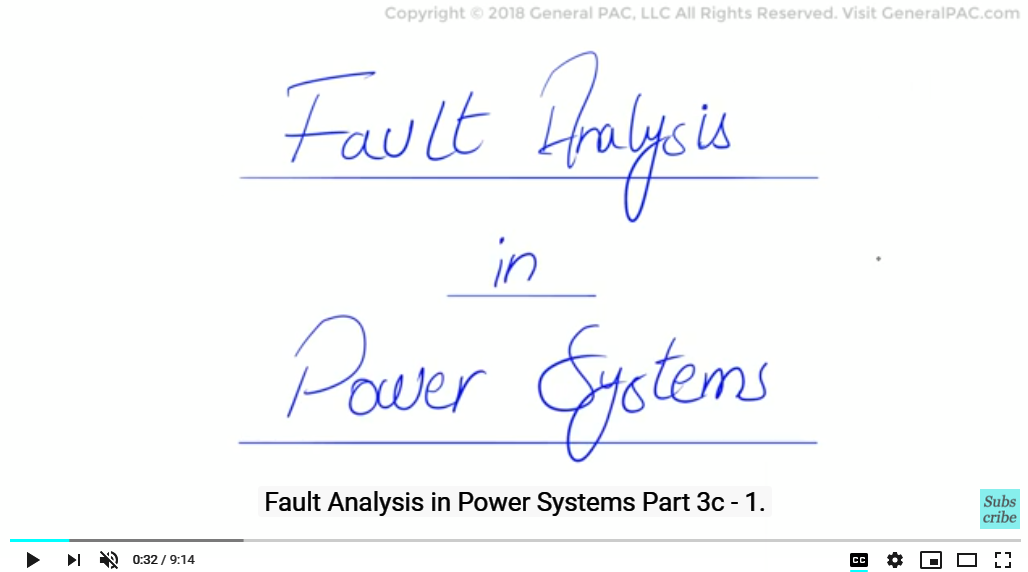Line to Line Faults, Calculations

Fault Analysis in Power Sytems Part 3c-1. In this series on fault analysis in power system, our objective is to hand calculate the current and voltage quantities for various fault.

In this video we will see how phase currents and voltage quantities are calculated for a line to line fault on line b & c on the 13.8kV side of the transformer.

So Let’s continue with our example which was introduced in the previous parts: We have a three phase synchronous generator having a short circuit capability of 600 MVAsc connected to a 115 kV bus. A delta – Wye transformer rated at 30 MVA connects the 115 kV bus to the 13.8 kV bus and has a positive and zero sequence impedance of 10 % at 30 MVA Step 1 of our calculation asks us to convert our system into perunit values and that is exactly what we did in part 2b of our series. Here we redraw the same network.

Before we draw the converted sequence network diagram, let’s take a look at the type of fault in hand. The phase to phase fault on the 13.8kV system should look something like this: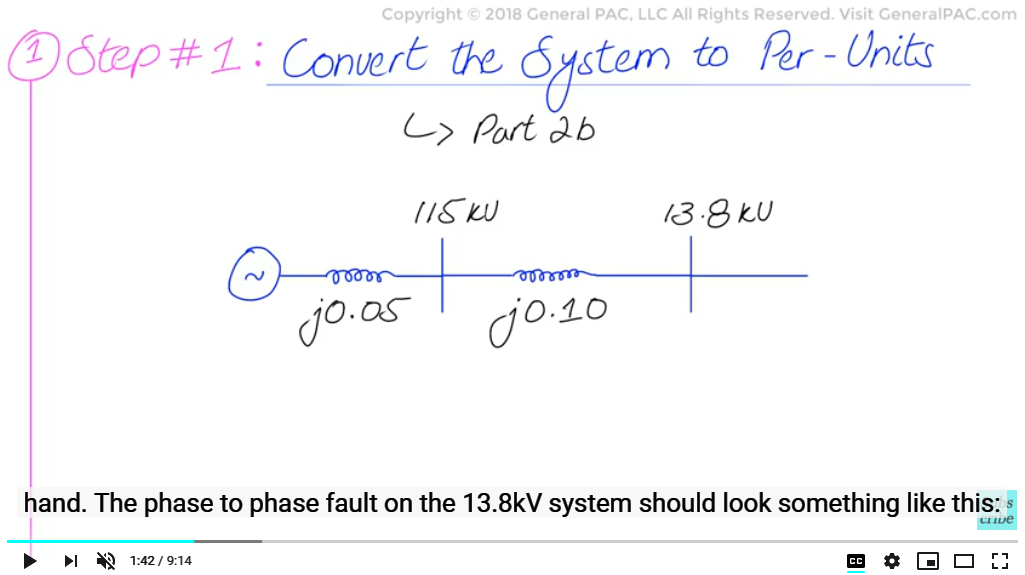Let us ignore the 1x and 2x current on the HV side and instead, focus on the LV side of the transformer. We have a line-to-line fault on the 13.8kV side in which phase b and c are involved. The fault current on “phase b” flows towards the point of the fault. However, on phase c, the fault current is flowing away from the point of the fault. Therefore, for a phase-to-phase fault, we should expect the fault current on both phases to be equal in magnitude but opposite in direction. Let’s us go through the hand calculations and verify this result.

Next we move to step 2, which asks us to identify the type of fault involved. As mentioned before, we have a line to line fault which is an unsymmetrical fault meaning an unbalanced fault. This type of fault produces sequence quantities positive and negative sequence components, current and voltage quantities, However, due to the nature of a line-to-line fault, it does not have a ground mode. Therefore, we should not expect any zero sequence current flowing for a line to line fault.

Next we move to step 3, which asks us to make sequence networks from the per unit converted system: So we draw the positive sequence network and we also draw the negative sequence network, again we don’t have a zero sequence network because we have a line to line fault which does not have a ground mode.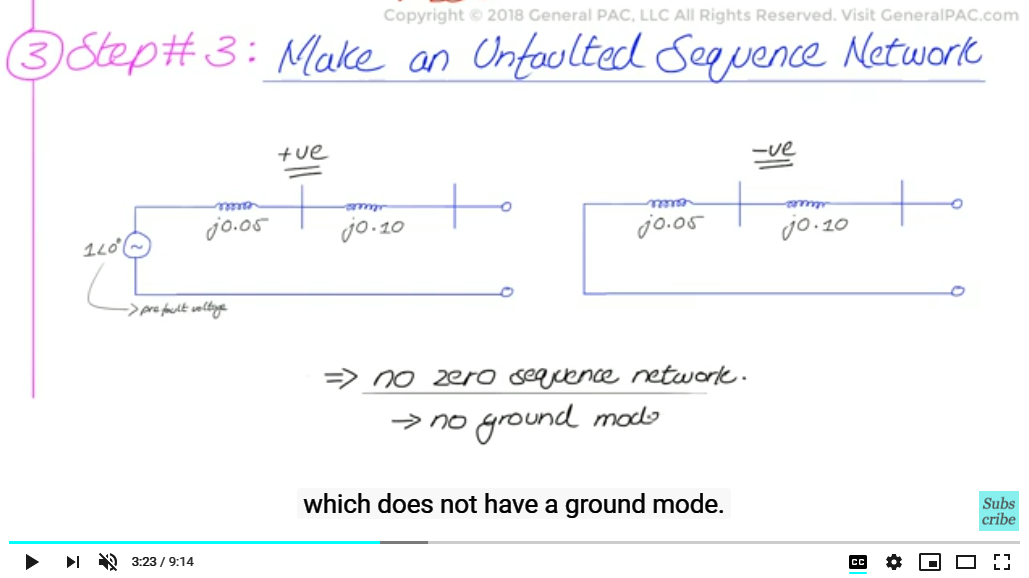And then, in Step 4, we will draw the faulted sequence network based on the type of fault we have. Now given that the fault is a line to line fault as we mentioned before we will connect the positive and negative sequence networks in parallel. This, we will discuss in great detail in our video on line to line fault in Topic 2: which is the Derivations of sequence network diagrams of our series but for now we just redraw the parallel connected faulted network.

To give a brief description of the faulted network, we basically have the positive sequence and negative sequence networks connected in parallel at the point of the fault. Line to line faults are faults that are although un-symmetrical, are not connected to ground which means that, zero sequence current will not be able to flow from ground into the fault, and this explains as to why zero sequence networks are omitted from the construction of the faulted sequence network, This also tells us that our subsequent calculations for finding fault currents and voltages quantities, zero sequence components will totally be ignored and considered zero.

So lets move toward step #5 and hand calculate the sequence current quantities. First, we set up a base value and then proceed to sequence current calculations:

The positive sequence current will be the fault current inside the loop Which can be found by dividing the voltage with the total impedance in the loop as follows:

The prefault voltage is 1 angle 0 and then the impedance in loop is the sum of positive and negative sequence impedances, that is, j0.05 + j0.10 + j0.10 + j0.05 per unit, and that gives us a value of –j3.333 per unit.

Now this is the positive sequence current that is flowing through the connected sequence network, that is flowing through the connected sequence network and this is the per unit value

Now we must calculate the current values in ampere so first we take the base value of power which is 30 MVA divided by the base value of voltage at the faulted end, 13.8kV which gives us a base value of current at 1255 Amperes. Multiply that base value of 1255 Amperes by the per unit and we actually get the ampere current that is flowing through the positive sequence network 4184 Amps at angle -90 degrees.

It is also evident from the faulted sequences network that the positive and negative sequence current will be equal in magnitude because they are the same currents in the loop, with the difference being that they have an opposite direction meaning they are flowing in opposite direction from each other.

To account for that we give a 180 degrees phase shift in the positive sequence current to find the negative sequence current So the negative sequence current simply equals 4184 amperes at the angle of +90 degrees instead of -90 degrees for the positive sequence.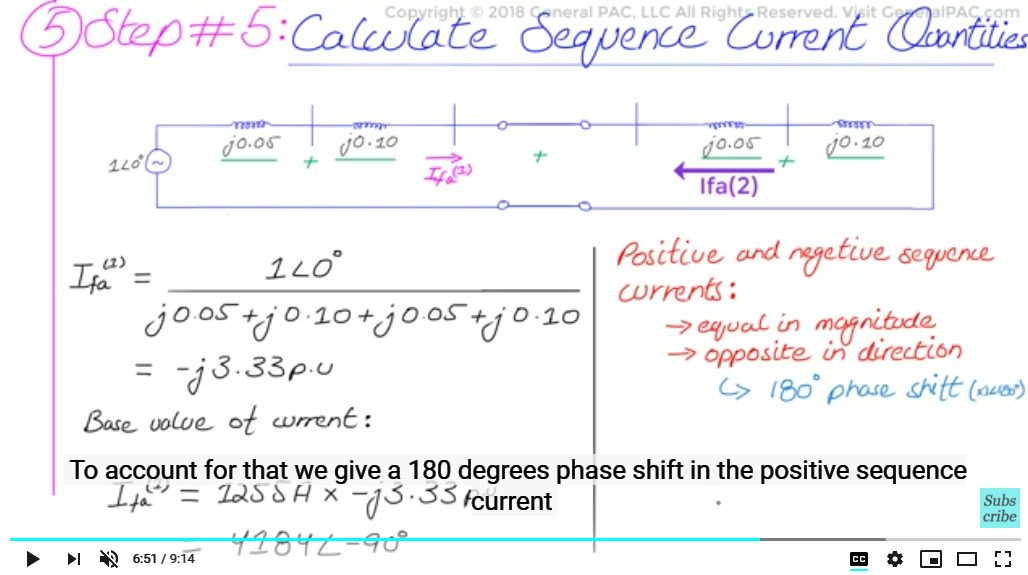So again negative sequence current equals 4184 at the angle of +90 degrees and as mentioned before, There will be no zero sequence component in a line to line fault.

Next, we move to step 6, to convert the sequence component values to phase current values, We have our old familiar set of three transformation equations:

Now, since there is no zero sequence current, they can be simplified even further to the following:

Now after plugging in the values of 1 angle 120 for a, 1 angle 240 for a squared operator, 4184 Amps angle -90 for positive sequence current and 4184 amps at angle of +90 for negative sequnce :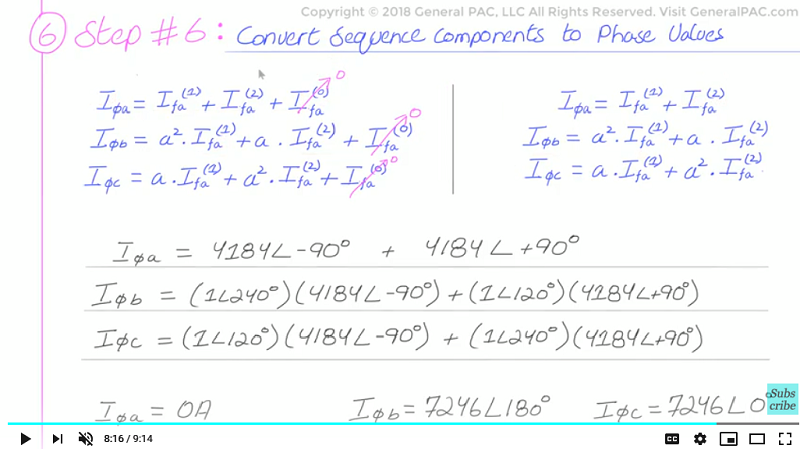What we finally get is the following:

We find that fault current in phase A is zero and fault current in phases B and C are equal and opposite of each other. This can be further explained intuitively by the fact that we assumed that phases B and C are shorted, hence there was no fault current in phase A because it was the unfaulted phase and does not carry any fault current. Phases B and C current quantities are the faulted phase and should be the same magnitude but opposite in direction which is evident by our hand calcluations. This matches well with the diagram that we drew earlier:

For part 3c-2, we will calculate the voltage quantities for a phase to phase fault. We hope that you find this tutorial valuable and useful as a professional or student please support us by becoming our patron and donating through patreon.com/Generalpac your generous donation will help us continue making high quality power system video tutorials. Thank You.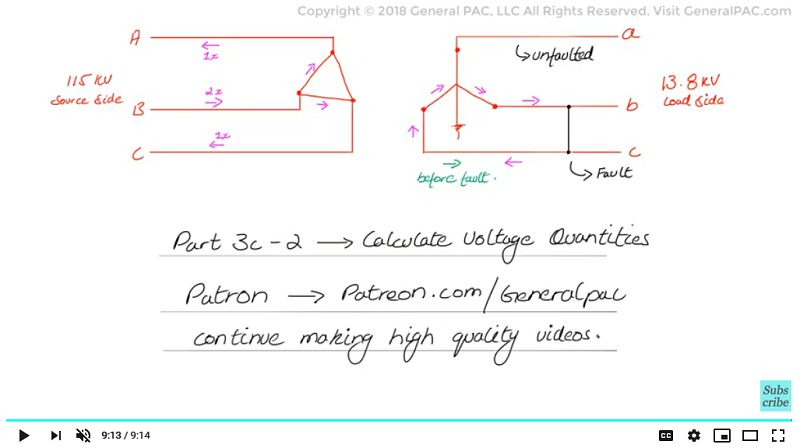Our coorporate sponsor AllumiaX,LLC published a new blog on "Fault Analysis in Power Systems". This blog covers the following topics:
• Types of Faults in Power System
• Steps to perform a Fault Analysis in a Power System
• Causes of Power System Faults#### Greetings from the GeneralPAC Team!

We make high-quality Power Systems Video Tutorials on complex topics that are free and open to everyone!  Thank you so much for supporting us through Patreon so we can continue doing good and valuable work.

What is Patreon and why do we use it?

Patreon is a fantastic portal that allows our fans and community to make monthly contribution (like Netflix subscription) so we can continue creating high-quality power systems video tutorials. In return, you get access to incredible perks like voting on future topics, getting your questions answered, access to VIP Q/A webinars with the creators of GeneralPAC, and much more! We THANK YOU for supporting us

Why do we need your support?

An incredible amount of time and effort is needed to develop high-quality video tutorials. Each video (Part 1 for example) takes approximately 10 hours to complete which includes learning the concept ourselves, brainstorming creative ways to teach and explain the concepts, writing the script, audio recording, video recording, and editing. It's no wonder why Hundreds-of-Thousands of people have watched, liked, subscribed, and left positive comments on Youtube channel. Your support truly makes all the difference.